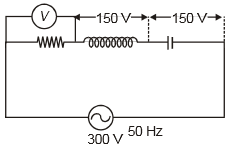You’ve reached the end of your free Videos limit.
#6 | AC Circuit containing only R
(Physics) > Alternating Current
Unable to watch the video, please try another server
Related Practice Questions :

In the circuit given below, the reading of the voltmeter V will be1.  0

2.  100 V

3.  200 V

4.  300 V

High Yielding Test Series + Question Bank - NEET 2020

Difficulty Level:

If the current in an AC circuit is given as $\mathrm{i}=\left(5\mathrm{A}\right)\mathrm{sin}\left(\mathrm{\omega t}-\frac{\mathrm{\pi }}{3}\right)$ when an emf $\mathrm{e}=\left(200\mathrm{V}\right)\mathrm{sin\omega t}$ is applied across the circuit. The circuit having component only

1.  L and C

2.  L and R

3.  C and R

4.  None of these

High Yielding Test Series + Question Bank - NEET 2020

Difficulty Level:

In a LCR series network, . The supply voltage is

1.  25V

2.  75V

3.  35V

4.  Zero

High Yielding Test Series + Question Bank - NEET 2020

Difficulty Level:

A 220 V, 50 Hz ac source is connected to an inductance of 0.2 H and a resistance of 20 ohms in series. What is the current in the circuit :

1.  10A

2.  5A

3.  33.3A

4.  3.33A

High Yielding Test Series + Question Bank - NEET 2020

Difficulty Level:

The potential difference V and the current i flowing through an instrument in an ac circuit of frequency f are given by $V=5\mathrm{cos}\omega \text{\hspace{0.17em}}t$ volts and I = 2 sin ωt amperes (where ω = 2πf). The power dissipated in the instrument is

(1) Zero

(2) 10 W

(3) 5 W

(4) 2.5 W